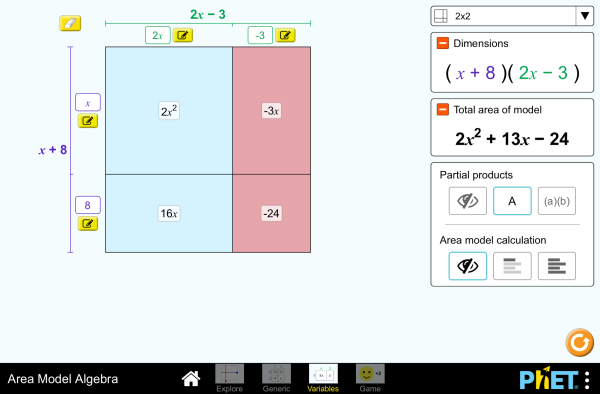# Area Model Algebra_面積模型與代數下載嵌入 關閉 嵌入模擬教學執行複本 利用 HTML 來嵌入模擬教學執行複本。您可以在 HTML 中改變已嵌入模擬教學的寬度及高度。 嵌入啓動模擬教學之圖樣

• Polynomials
• Factors
• Products

### 學習目標

• Develop and justify a method to use the area model to determine the product of a monomial and a binomial or the product of two binomials.
• Factor an expression, including expressions containing a variable.
• Recognize that area represents the product of two numbers and is additive.
• Represent a multiplication problem as the area of a rectangle, proportionally or using generic area.
• Develop and justify a strategy to determine the product of two multi-digit numbers by representing the product as an area or the sum of areas.

### 標準對齊

#### 共用核心 - 數學

3.MD.C.7
Relate area to the operations of multiplication and addition.
3.MD.C.7c
Use tiling to show in a concrete case that the area of a rectangle with whole-number side lengths a and b + c is the sum of a × b and a × c. Use area models to represent the distributive property in mathematical reasoning.
3.MD.C.7d
Recognize area as additive. Find areas of rectilinear figures by decomposing them into non-overlapping rectangles and adding the areas of the non-overlapping parts, applying this technique to solve real world problems.
4.NBT.B.5
Multiply a whole number of up to four digits by a one-digit whole number, and multiply two two-digit numbers, using strategies based on place value and the properties of operations. Illustrate and explain the calculation by using equations, rectangular arrays, and/or area models.
6.EE.A.3
Apply the properties of operations to generate equivalent expressions. For example, apply the distributive property to the expression 3 (2 + x) to produce the equivalent expression 6 + 3x; apply the distributive property to the expression 24x + 18y to produce the equivalent expression 6 (4x + 3y); apply properties of operations to y + y + y to produce the equivalent expression 3y.
6.EE.A.4
Identify when two expressions are equivalent (i.e., when the two expressions name the same number regardless of which value is substituted into them). For example, the expressions y + y + y and 3y are equivalent because they name the same number regardless of which number y stands for..
6.NS.B.4
Find the greatest common factor of two whole numbers less than or equal to 100 and the least common multiple of two whole numbers less than or equal to 12. Use the distributive property to express a sum of two whole numbers 1-100 with a common factor as a multiple of a sum of two whole numbers with no common factor. For example, express 36 + 8 as 4 (9 + 2)..
7.EE.A.1
Apply properties of operations as strategies to add, subtract, factor, and expand linear expressions with rational coefficients.
7.NS.A.2c
Apply properties of operations as strategies to multiply and divide rational numbers.
8.EE.C.7b
Solve linear equations with rational number coefficients, including equations whose solutions require expanding expressions using the distributive property and collecting like terms.

### 教學提示模擬教學的控制概觀、模型簡化與引導學生思考 ( PDF ).

### 老師提供的活動

Expandiendo y Factorizando Expresiones AlgebraicasAmanda McGarry (Traducción de Mayra Tavares) 國中 指引 數學
Games Remote Lesson ideasTrish Loeblein 國中

K-5

Remote

Expanding and Factoring Algebraic ExpressionsAmanda McGarry 國中 指引 數學
Algebraic ExpressionsAmanda McGarry 國中 指引 數學
Expresiones AlgebraicasAmanda McGarry (Traducción por Mayra Tavares) 國中 指引 數學
Exploring Factoring as Undoing the Distributive PropertySarah Hampton 高中

SECUNDARIA: Alineación PhET con programas de la SEP México (2011 y 2017)Diana López 國中

PREPARATORIA: Alineación de PhET con programas de la DGB México (2017)Diana López 高中

Middle School Math Sim AlignmentAmanda McGarry 國中 其它 數學
Using the area model with expressionsJennifer Knudsen, Teresa Lara-Meloy, Amanda McGarry 國中 討論 數學
Usando el Modelo de Áreas con Expresiones Jennifer Knudsen, Teresa Lara-Meloy, Amanda McGarry (Traducción Mayra Tavares) 國中 討論 數學
Modelo de Áreas, Cambio de Expresiones Andrés Gualberto Palomo Cuevas 高中 Remote

projectile motion alya qasem 大學-簡介 實驗室 物理
Would You Rather ... Karen Gann 高中 指引 數學
Area Model Algebra - Multiplying Polynomials Carolyn Felknor 國中 指引 數學
ARTICULANDO ÁLGEBRA E GEOMETRIA: O CASO DAS EQUAÇÕES ALGÉBRICAS NO ESTUDO DE ÁREAS Verusca Batista Alves; Ana Carolina Costa Pereira 國中 實驗室

Distributive Property Review Tim Kissinger and Larry Peters 國中 討論

Serbian 全部 СрпскиМатематика преко површина - Алгебра
Sinhalese 全部 Sinhaleseවර්ගඵල ආකෘති වීජ ගණිතය
Tamil 全部 Tamilமாதிரி உருவின் எண்(அட்சர) கணிதம்

HTML5 sims can run on iPads and Chromebooks, as well as PC, Mac, and Linux systems.

iOS 12+ Safari

Android:
Not officially supported. If you are using the HTML5 sims on Android, we recommend using the latest version of Google Chrome.

Chromebook:
The HTML5 and Flash PhET sims are supported on all Chromebooks.
Chromebook compatible sims

Windows Systems:

Macintosh Systems:

Linux Systems:

• Jonathan Olson (developer)
• Karina Hensberry
• Susan Miller
• Ariel Paul
• Kathy Perkins
• Mariah Hermsmeyer (artwork)
• Diana López Tavares (artwork)
• almond-0.2.9.js
• base64-js-1.2.0.js
• FileSaver-b8054a2.js
• font-awesome-4.5.0
• game-up-camera-1.0.0.js
• he-1.1.1.js
• himalaya-0.2.7.js
• jama-1.0.2
• jquery-2.1.0.js
• lodash-4.17.4.js
• pegjs-0.7.0.js
• seedrandom-2.4.2.js
• text-2.0.12.js
• TextEncoderLite-3c9f6f0.js